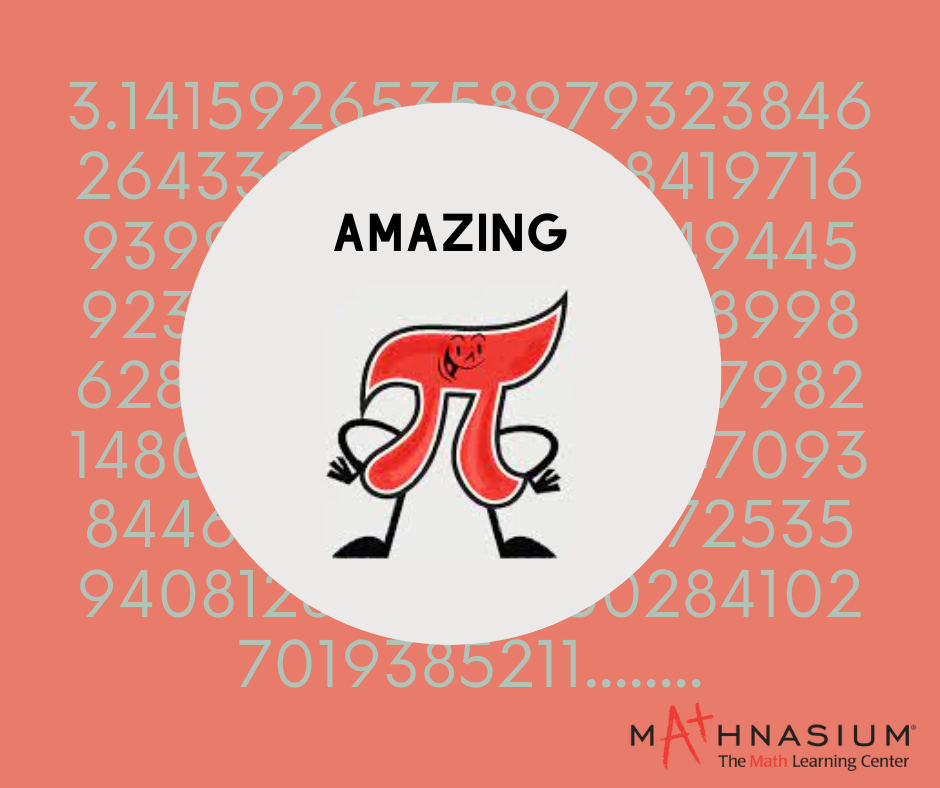877-601-6284
Get Started by Finding a Local Center

# Amazing Pi fun facts

Mar 14, 2023 | Location Cherry HillPi (π) is one of the most fascinating and mysterious mathematical constants in the world. Defined as the ratio of the circumference of a circle to its diameter, pi has captivated mathematicians and scientists for thousands of years. In this blog, we’ll explore some amazing facts about pi, including its history, properties, and some fun facts that you might not have heard before.

Pi is an irrational number Pi is an irrational number, which means that it cannot be expressed as a finite decimal or fraction. Its decimal representation goes on forever, without repeating. This property has intrigued mathematicians for centuries, and many have attempted to calculate pi to as many digits as possible. As of 2021, the most accurate calculation of pi has been done up to 62.8 trillion digits!

Pi is transcendental Not only is pi irrational, but it is also a transcendental number. This means that pi is not a root of any non-zero polynomial equation with rational coefficients. In simpler terms, it cannot be expressed as the solution to any algebraic equation with rational coefficients. This property makes pi even more mysterious and intriguing.

Pi has been known since ancient times The first recorded approximation of pi was done by the ancient Egyptians around 1650 BC. They estimated the value of pi to be around 3.16. The ancient Babylonians also had a similar approximation, as did the ancient Greeks. The Greek mathematician Archimedes is credited with being the first to accurately calculate pi using a geometric method around 250 BC.

Pi is an infinite and non-repeating decimal Pi is an infinite decimal that does not repeat. This means that there is no pattern to the digits that follow the decimal point. The digits of pi have been calculated to trillions of decimal places, and there is no evidence of a pattern emerging. This property of pi has fascinated mathematicians for centuries, and many have attempted to find patterns in the digits of pi.

Pi is a universal constant Pi is not only a mathematical constant but also a universal one. It appears in many different areas of mathematics and science, including trigonometry, calculus, and geometry. It is also used in physics to calculate the circumference and area of circles, spheres, and other curved shapes.

Pi has been used to break world records The calculation of pi to as many digits as possible has been a popular pastime for mathematicians and computer enthusiasts. In 2019, Emma Haruka Iwao, a Google employee, broke the world record for the most accurate calculation of pi by computing it to 31.4 trillion decimal places using Google Cloud technology.

Pi Day is celebrated on March 14th Pi Day is an annual celebration of pi that takes place on March 14th (3/14), which is a reference to the first three digits of pi. The first Pi Day was celebrated in 1988 by physicist Larry Shaw at the San Francisco Exploratorium. Since then, it has become a popular holiday for math enthusiasts around the world.

The symbol for pi has an interesting history The symbol for pi (π) was first used by Welsh mathematician William Jones in 1706. However, it was not widely adopted until the 1730s when Swiss mathematician Johann Lambert started using it. The symbol was chosen because it is the first letter of the Greek word for perimeter (περίμετρος).

Pi has been used to hide secret messages Pi has been used to hide secret messages in the past. In World War II, the Allies used pi to encode messages sent between the US and the UK. They would use the digits of pi to represent letters, numbers, and other characters

In conclusion, pi is a truly amazing number that has captured the hearts and minds of mathematicians and math enthusiasts around the world. From its infinite and non-repeating decimal to its use in all sorts of equations and applications, pi is truly a wonder of the mathematical world. And with fun facts like Pi Day and pi-inspired jokes and puns, it's also a number that can bring a smile to your face. So the next time you see a circle or a mathematical equation, remember the amazing and mysterious number that is pi!

##### Locations near
Could not find Center, try again